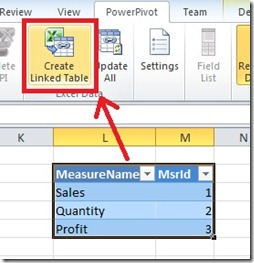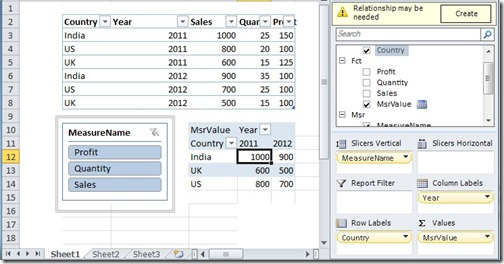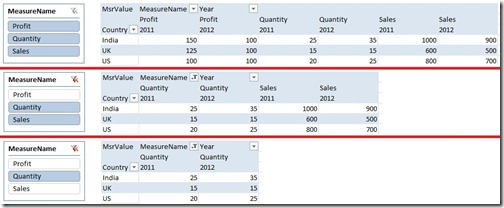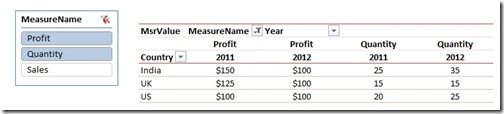# Measure Selection using Slicers in PowerPivot

As a Business Intelligence professional, I find it really fascin(/frustr)ating working with Excel users. You get exposed to a completely different way of thinking when you work with them, not to say that they are extremely smart and demanding. From the moment I started working with PowerPivot and interacting with Excel users, I knew it was not going to be easy tackling their questions and requirements. You simply cant escape by saying that the required feature is not available because they will push you till you find a workaround. This was the case when one of my users came back to me asking for a slicer which will dynamically help them to select the measures. I replied saying that the slicers are not intended for that purpose and should be used for filtering the data (and not for just selecting or deselecting values like Measures, which can be easily done from the Field List pane). However, the user pushed back saying that he would like to create a dashboard for the top management and didn’t want them to be using the Field List pane. That is when I had to put on my thinking cap.For the purpose of this post, I am going to use some simple mocked up data which involves three measures (Sales, Quantity and Profit) for country and year.Follow the steps below to recreate a slicer which can be used to select or deselect the measures in a pivot table:- 1) Create a table which will have the list of measure names needed in the slicer in the MeasureName column and their representative integer ids in the MsrId column. Import this table into PowerPivot using Linked Tables and name it as Msr.There is no need to make any relations with the existing tables, and Msr table should be a standalone table. 2) Now make a new measure called MsrValue with the formula given below MsrValue:=switch (TRUE,
Min ( Msr[MsrId] ) = 1,
Sum ( Fct[Sales] ),
Min ( Msr[MsrId] ) = 2,
Sum ( Fct[Quantity] ),
Min ( Msr[MsrId] ) = 3,
Sum ( Fct[Profit] )
)This measure will check the value of the MsrId column, and then use the appropriate measure. However, it should be noted that when there are more than one value of MsrId (or if more than one measures are selected), only the measure having minimum value of MsrId would be displayed. So how will we display more than one measures on the pivot table? Read on. 3) With this very simple setup, we are ready to view the results in the Pivot table. Drag and drop MeasureName column into the Slicer, Year into the Column Labels and Country in the Row Labels. Then drag and drop MsrValue in the Values and we should have something similar to the image below. I have also included the fact rows above the pivot so that we can compare.Now there are a couple of problems with this pivot table. You would have noticed that the pivot table works correctly if only one measure is selected, but if there are more than one selections for the measure name, it will only show the measure with the minimum of the MsrId (eg, Sales in the image above). Also, we would like to see the name of the measure in the column above for clarity. To solve both of these problems, follow the next step. 4) Just drag and drop the MeasureName column in the Column Labels above the Year.Now you can see that multiple measures are displayed and also that the measure names are displayed in the column above.You can also change the formatting of the measures if needed MsrValue:=switch (TRUE,
Min ( Msr[MsrId] ) = 1,
FORMAT ( Sum ( Fct[Sales] ), “\$#,#0” ),
Min ( Msr[MsrId] ) = 2,
format ( Sum ( Fct[Quantity] ), “#,#0” ),
Min ( Msr[MsrId] ) = 3,
format ( Sum ( Fct[Profit] ), “\$#,#0” )
)Luckily, that wasn’t as hard as I thought (which means that I could still use my evening free time to focus on more important problems like whether the chicken or egg came first into the world!). If you don’t have such important problems to focus on like me, you can download the completed file from this link and test it out on your own!### Posted by SQLJasonpng

Technique does not seem to be working when file converted to Excel 2013.SQL_Jason

Check out this post – http://www.sqljason.com/2012/11/changing-pivottable-names-in-excel-2013.html
You must be using the Excel Preview version like me, and looks like it doesn't handle the FORMAT function inside the measure.Bob Phillips

@png,

Which part is giving you problems? I just recreated it in 2013 and it works fine as far as I can see.png

Used this technique on a PowerPivot table with < 200 rows (but a lot of columns). Included 17 measures in the SWITCH function. When added to a slicer, one selection worked fine but with a multiple selection, it choked on my 32-bit system. Apparently, the memory requirements for this are very high.Kevin Burrus

Does anyone know if there is an alternative if using PowerPivot 2008R2? The switch command does not seem to be available. We are currently stuck on PowerPivot 2008R2 until we can get SharePoint updated. I would appreciate thoughts- If I get an answer I'll loop everyone in!Kevin Burrus

Looks like I just answered my own question. I have the case where I only need to have one measure or the other, without having to worry about 2 concurrent selections. This works for me:

IF (Min ( Msr[MsrId] ) = 1,Sum ( Fct[Sales] ),IF(Min ( Msr[MsrId] ) = 2,Sum ( Fct[Quantity] ),Sum ( Fct[Profit] )))Peter Wickwire

I am extremely interested in using this technique to help make dashboards able to display a number of different measures in Fixed amount of space. I have done my best to use your code to create a "slicer driven" measure selection in a model that contains a number of measures all displaying different calculations of web site "hits" (I use the term Impressions.

My disconnected measure table is called MSR — here's what it contains:
Measures Msrid
Available Imps 1
Billable Imps 2
CPM 3
Direct Sold Imps 1P DFP 4
Direct Sold Imps 3P 5
House Imps 1P DFP 6
Ordered Imps 7
Remnant Imps 1P DFP 8
Remnant Imps 3P 9
Reported Imps 3P 10
Stated Imps 1P 11
Billable Imps Prv Period 12
Billable Imps Same Prd Prev Yr 13

Here is the code for my calculated measure: Note that I am simply reusing measures that I've created already instead of re-writing each measure calculated measure in the formula.

=Switch (TRUE,
Min( Msr[Msrid] )=1,
[Available Imps],
Min( Msr[Msrid] )=2,
[Billable Imps],
Min( Msr[Msrid] )=3,
[CPM],
Min( Msr[Msrid] )=4,
[Direct Sold Imps 1P DFP],
Min( Msr[Msrid] )=5,
[Direct Sold Imps 3P],
Min( Msr[Msrid] )=6,
[House Imps 1P DFP],
Min( Msr[Msrid] )=7,
[Ordered Imps],
Min( Msr[Msrid] )=8,
[Remnant Imps 1P DFP],
Min( Msr[Msrid] )=9,
[Remnant Imps 3P],
Min( Msr[Msrid] )=10,
[Reported Imps 3P],
Min( Msr[Msrid] )=11,
[Stated Imps 1P],
Min( Msr[Msrid] )=12,
[Billable Imps Prv Period],
Min( Msr[Msrid] )=13,
[Billable Imps Same Prd Prev Yr])

My issue is that the actual numbers displayed by the measures shown using the "selected formula" noted above, is different from what those same measures display when they are used independently. Worse, the independently placed measures show considerably more data. For example, I have values of the "independent measures for each month of the year. But, the measure values displayed through the selector process only shows 4 or 5 months.

The "measure driven" values are always higher than the independent measures by themselves, but I can't see any other pattern. I have tried two different version of the formula above — one used the calculated measure names themselves. The other used the formulas themselves.

Also, none of the calculations are complex. THey are all simply sums or averages.

Any Suggestions as I REALLY want to use this process.

Peter Wickwire:
prwindenver@gmail.comSQL_Jason

Would it be possible to share your powerpivot file to jason143@gmail.com so that I can take a look?Matt D

Just wondering if there was a solution for this?

I want to change the measures in the chart not values.

If i use measures to change how the chart is split i can either sum all values related, this first, the last. How can i see all measure categories?

For example. Looking at value by year. Instead, split the values by something like age band, or their first payment method. I’ve solved this before by making a number of bookmarks but means you have to create all the charts first instead of a dynamic solutions.

Thanks so muchRyan Stearns

This worked great thanks!

One small question is when I use this it doesnt filter other criteria down to those that have values. For example I am showing Gr Hrs by plant, filtered by year from another slicer. When I use the regular measure, it will only show plants that have hours for the given year, but when i use the switch formula, it shows all plants that have ever had hours, with the value being blank.

Any way around this?James Harper

I get this as well, did anyone find out if it is possible to retain the other slicer filters?James Harper

I get this as well, did anyone find out if it is possible to retain the other slicer filters?Shawn

Hi- i have this same issue as well, in that once the FORMAT() function is applied, i get blank rows. Any work around would be appreciated..Mark Hodge

Aware this post is very old but really like this idea. When I implemented it (in Excel 2010) I got everything to work as I wanted, EXCEPT that the FORMAT command you used meant my table returned text values, rather than useable currency/percentage values. As I wanted to chart the outcomes this was no good to me. IS there anything I can tweak to return useable currency/percentage values?SQL_Jason

Just thinking out aloud here, can't we use the measure without format function and then format it in the chart directly?Mark Hodge

Absolutely could, but wanted to offer user chance to select measure (varying between currency and %) and have the graph scale automatically adjust to either \$ or %. Thanks anyway for your response though.Shawn

Really appreciate this solution. Like I believe has already been mentioned, I seem to have a issue that when the FORMAT() function is applied, i get BLANK rows that don't show up otherwise. Is there a work around for this?

Thanks again.. you all are way smarter than me with DAX

[…] of this demo, let us use the PowerPivot file that I created in Excel 2010 for my last post – Measure Selection using Slicers in PowerPivot. You can download it from this link. If you open it in Excel 2010, you can see the original table […]

[…] couple of months back, I had written a post on ‘Measure selection using Slicers in PowerPivot’ and this turned out to be one of my most popular ones in the PowerPivot / DAX category. It was […]Nicholas Osman

Hi,

I am trying to do the same as yourself. However, mine does not want to work with a formatting included.

This one works;

Measure 2:=SWITCH( TRUE,
Min ( Table2[Msrld] ) = 1,
Sum ( NTR_Row_Data[SUMQuantity] ),
Min ( Table2[Msrld] ) = 2,
AVERAGE( NTR_Row_Data[SUMCostOfSale] ),
Min ( Table2[Msrld] ) = 3,
Sum ( NTR_Row_Data[SUMNettValue] ),
Min ( Table2[Msrld] ) = 4,
AVERAGE ( NTR_Row_Data[GM] ))

When l try to format the calculation depending on selection, this generates an error. The formats that l want are;

Measure 2:=SWITCH( TRUE,
Min ( Table2[Msrld] ) = 1,
FORMAT( Sum ( NTR_Row_Data[SUMQuantity] ),”#,#0″),
Min ( Table2[Msrld] ) = 2,
FORMAT( AVERAGE( NTR_Row_Data[SUMCostOfSale] ),”\$#,#0″),
Min ( Table2[Msrld] ) = 3,
FORMAT( Sum ( NTR_Row_Data[SUMNettValue] ),”\$#,#0″),
Min ( Table2[Msrld] ) = 4,
FORMAT( AVERAGE ( NTR_Row_Data[GM] )”%”))

I have generated the correct format for one of the selections;

Measure 3:=switch (TRUE,
Min ( Table2[Msrld] ) = 1,
FORMAT( Sum ( NTR_Row_Data[SUMQuantity] ),”\$#,#0″))

This works but not if there is more that one of them.

Kind regards,Nicholas Osman

Hi,

I have a measure;

Measure 2:=SWITCH( TRUE,
Min ( Table2[Msrld] ) = 1,
Sum ( NTR_Row_Data[SUMQuantity] ),
Min ( Table2[Msrld] ) = 2,
AVERAGE( NTR_Row_Data[SUMCostOfSale] ),
Min ( Table2[Msrld] ) = 3,
Sum ( NTR_Row_Data[SUMNettValue] ),
Min ( Table2[Msrld] ) = 4,
AVERAGE ( NTR_Row_Data[GM] ))

This works with a slicer selection. The only issue is that the format is incorrect for some of the selections e.g. GM is Gross Margin as a %. The required format is

Measure 2:=SWITCH( TRUE,
Min ( Table2[Msrld] ) = 1,
FORMAT( Sum ( NTR_Row_Data[SUMQuantity] ),”#,#0″),
Min ( Table2[Msrld] ) = 2,
FORMAT( AVERAGE( NTR_Row_Data[SUMCostOfSale] ),”\$#,#0″),
Min ( Table2[Msrld] ) = 3,
FORMAT( Sum ( NTR_Row_Data[SUMNettValue] ),”\$#,#0″),
Min ( Table2[Msrld] ) = 4,
FORMAT( AVERAGE ( NTR_Row_Data[GM] )”%”))

However this does not work. however, this one below does

Measure 3:=switch (TRUE,
Min ( Table2[Msrld] ) = 1,
FORMAT( Sum ( NTR_Row_Data[SUMQuantity] ),”\$#,#0″))Lana

WHAT A LIFE SAVER, thank you for this. I was just about to VBA it all when I found this blog, really grateful.Lana

Forgot to mention a fantastic thing about it. I have loads of measures in Power Pivot. This solution allowed me to “hide from client” my entire sales data including calculated measures, with the only thing elegantly showing in the pivots field list being “Measures” ! 🙂 Thanks again!Alison

Has anyone had any experience doing a similar thing in POWERBI?SQLJason

The same approach should work. Create a calculated table (or just use the Enter data feature in Power BI) to create a list of the measure names. And then use the same technique basically.Raj

Excellent Work Buddy.. solution works perfectly.todd

I realize this is an old post, but this approach worked great for me. One additional question… Is there a way to calculate a variance in your example above. For example, the variance between 2011 and 2012 for both Quantity and Profit in your example above?SQLJason

Sure, you can make 2 additional rows for Quantity Variance and Profit Variance. Also, make sure you make measures that give you the required variance, and then just add those measures into MsrValuetodd

I tried that, but it results in multiple columns of same data.
My table has a version (act, plan, ly) in a single column going down the rows, and my accounts (Sales Qty, Dollars, Margin) in each column.
So, when I try to calculate a difference for Sales Qty for act vs. plan, I get three columns showing the same data in the Act, Plan, LY columns. I would like this to look a bit cleaner for the users. (I apologize, but I am a beginner at this.)SQLJason

send me an email to jason143@gmail.com, with some sample data and your measure expression. Also, put a picture on how you want the end result to look. The better the detail, the easier it makes my life and the faster you get a response 🙂Charles Philpott

Thank you for the solution you’ve posted. It appears to be the gift that keeps on giving even 8 years later. I’ve applied the above technique to switch between reported BPD, GPD, SalesPD, Barrels, Gallon, and Sales. It works great. The challenge I’m having is getting the Pivot detail. When selecting on any of those values, double-clicking on a displays amount/quantity will display all the record detail making up that number. When I do this with the measure, it retrieves just one record with the measure number. Any thoughts on how to work around this? Thanks.

This site uses Akismet to reduce spam. Learn how your comment data is processed.